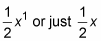##### Differential Equations For Dummies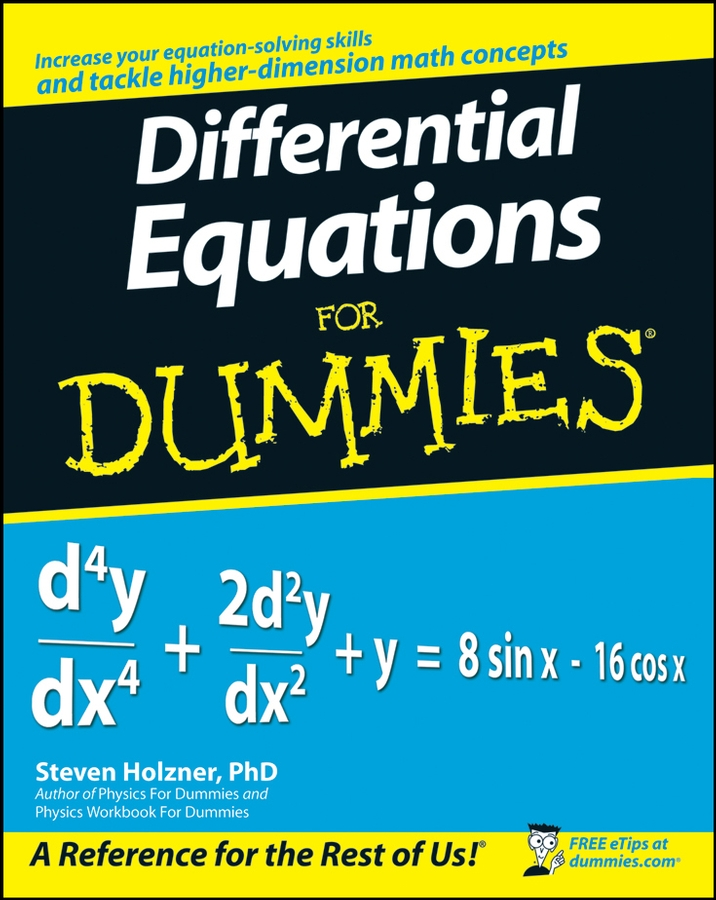Calculus is the mathematics of change — so you need to know how to find the derivative of a parabola, which is a curve with a constantly changing slope.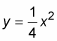The figure below shows the graph of the above parabola.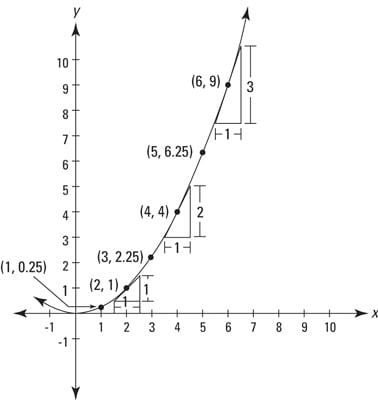Notice how the parabola gets steeper and steeper as you go to the right. You can see from the graph that at the point (2, 1), the slope is 1; at (4, 4), the slope is 2; at (6, 9), the slope is 3, and so on.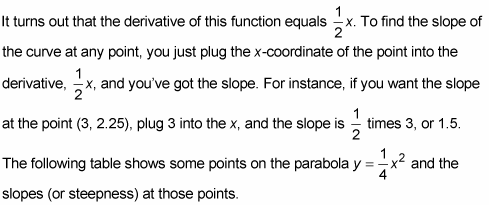x (horizontal position) 1 2 3 4 5 6 etc. y (height) 0.25 1 2.25 4 6.25 9 etc. 1/2 x (slope) 0.5 1 1.5 2 2.5 3 etc.

Here’s the calculus. You write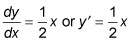And you say,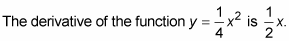Or you can say,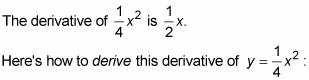1. Take the power and put it in front of the coefficient.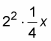2. Multiply.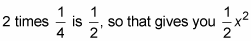(Note that this is only a temporary, interim result on the road to the solution below; by itself, it is meaningless.)

3. Reduce the power by 1.

In this example, the 2 becomes a 1. So the derivative is